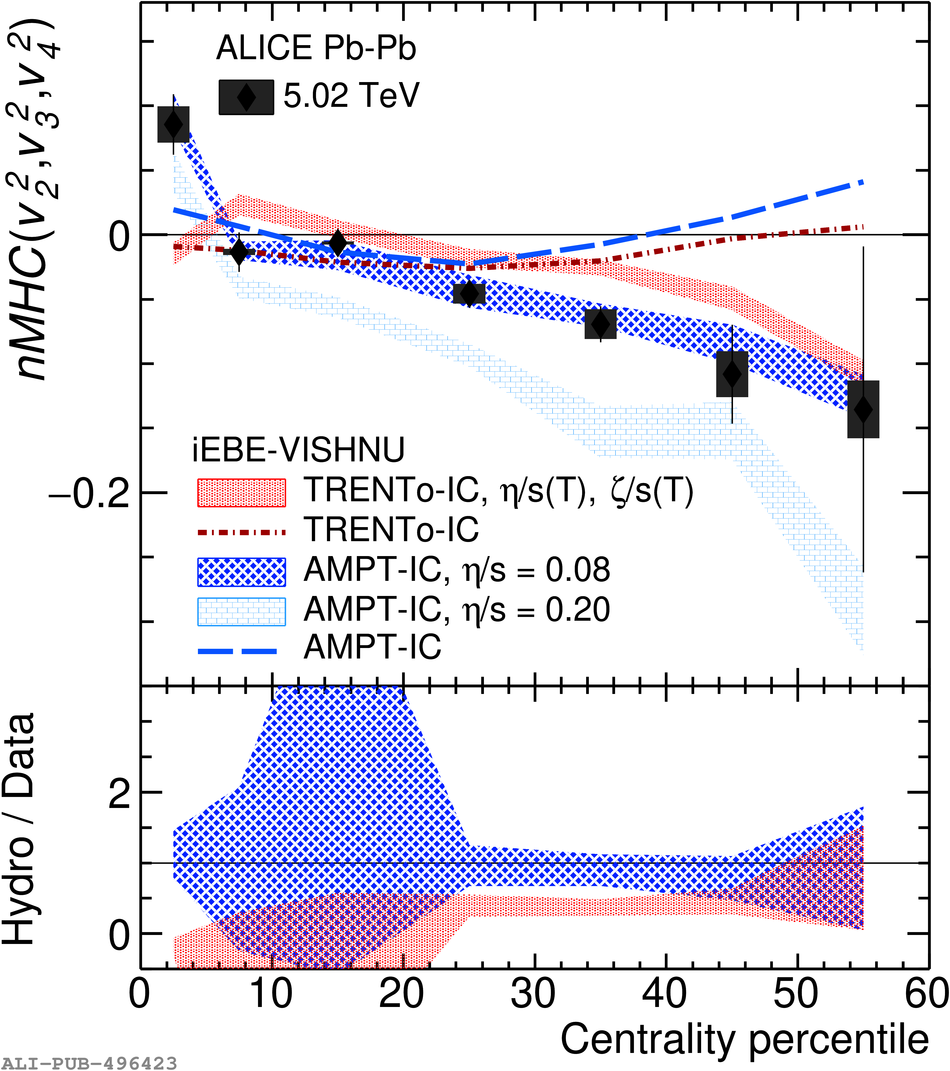# Figure 3

 Centrality dependence of $nMHC(v_{3}^{2}, v_{4}^{2})$ and $nMHC(v_{2}^{2}, v_{3}^{2}, v_{4}^{2})$ in \PbPb\ collisions at $\sqrt{s_{\rm NN}} = 5.02$ TeV (solid markers) and 2.76 TeV (open markers). Statistical uncertainties are shown as vertical bars and systematic uncertainties as filled boxes. Data points are shifted for visibility.